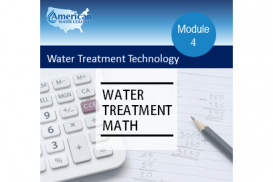Water Treatment Technology Certificate Program
Water Treatment Math
This course is designed to acquaint students with the math, formulas, and calculations used in water treatment, storage and distribution. Course material consists of reading assignments, video lectures, review questions, study problems, and lesson...
View more
Course content
• Powers and Scientific Notation
• Video Lecture
• Assignment
• Powers and Scientific Notation Quiz
• Dimensional Analysis
• Rounding and Estimating
• Solving for the Unknown Value
• Ratios and Proportions
• Averages
• Percent
• Linear Measurements
• Area Measurements
• Volume Measurements
• Conversions
• Graphs and Tables
• Per Capita Water Use
• Domestic Water Use Based on Household Fixture Rates
• Water Use per Unit of Industrial Product Produced
• Average Daily Flow
• Surface Overflow Rate
• Weir Overflow Rate